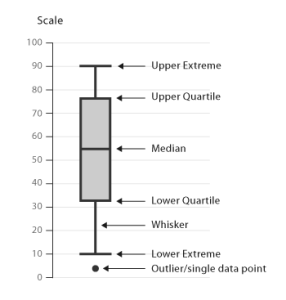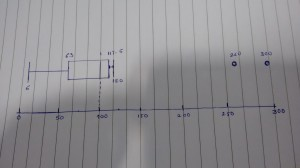# Understanding Box and Whisker Plots

#### Posted on October 27, 2014 by By Shraddha Tambe, in Business Intelligence, Data Visualization | 0

Understanding Box and Whisker PlotsA box plot or a Box-and-Whisker plot is one of the graphical methods to represent data distribution, very much like our beloved histograms. It helps understand the variations in a sample of data. It essentially displays the distribution of data based on a five number summary: minimum, lower quartile, median, upper quartile, and maximum.

In the set of data points,
Minimum value, as its name suggests, is the smallest value in the data set
Maximum value is the largest value in the dataset
Median value is value of the data point at the middle of the data set. E.g. if your sample has 15 data points, value of the 8th data point would be the median. However, in case there are 16 values, the median would be an average of the 8th and 9th data points.
Lower Quartile is actually a median value of the lower half of the data points
Upper Quartile is a median value of the upper half of the data points

The whiskers are drawn at the minimum and maximum value and represent the full range of data, whereas the range between the upper and lower quartiles, the IQR (Inter Quartile Range) represents the likely variation of the data.

We could see some other values in the figure alongside, marked as outliers. Outliers are like surprisingly high maximums or surprisingly low minimums in a dataset, which are not very uncommon. Eliminating outliers before calculating the summary values is essential to get more accurate insights into the data set. One way of identifying outliers which is commonly used is to consider all the data points more or less than 1.5 times the upper or lower quartiles as outliers.

The Box and Whisker plot shows more than just four groups of the data. It helps us see which way the data sways, like are the values clustered towards the median? Or do they cluster towards the max and min with sparse data at the middle. The size of the box, the position of the median in the box, the length of the whiskers gives us an overall picture of the skews in the data.Though comparing box plots with histograms is the not the intent of this blog, I have put in the figure here to just help better understanding. The figure shows different types of data distributions and how that is represented in histograms and box plots. As the histogram displays the actual data points in the data set (or sometimes grouped data points for larger datasets), it could provide greater insights when variation in the data set is high.

One advantage of box plots is that we could plot parallel Box plots for different datasets and have a comparative view of the variations across datasets. For example, we have collected information for daily sales information for different product lines. We can plot box and whisker plots for each of these product lines in parallel to comparatively analyze the variations in the sales data.

Example

Now, I am going to take a small sample of data points and plot the box plot for the same –

Suppose we have sample of monthly sales figures for a product from Jan to Dec as {50,76,115,80,9,6,100,120,100,100,300,260}

First Step is to sort the data à {6,9,50,76,80,100,100,100,115,120,260,300}

Below are the calculated summary values: (We have even number of values)

```Median = (100 + 100)/2 = 100

Lower Quartile = (50 + 76)/2 = 63

Upper Quartile = (115+120)/2 = 117.5

IQR = (LQ-UQ) = 117.5-63 = 54.5

For calculating outliers, we will consider all values that are either lower than LQ-(IQR*1.5) or higher than UQ+(IQR*1.5).

LQ-(IQR*1.5) = -18.75

UQ+(IQR*1.5) = 199.25

As per above, outliers are 260 and 300.```

As per our calculations,this is how the box plot looks like –In the same example, if we have monthly sales information for various products, we could plot parallel box plots for each product to gain a comparative view which could also provide useful business insights.

Helical IT SolutionsBest Open Source Business Intelligence Software Helical Insight is Here